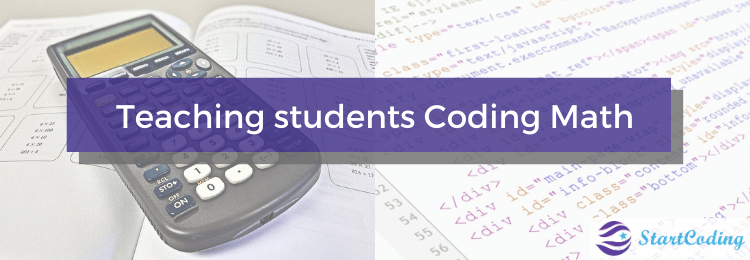StartCoding# Teaching students Coding Math

Coding is a hands-on approach to teach mathematical thinking. When students create or debug a program, they practice solving problems and develop algorithmic and computational thinking. Techniques of breaking down and analyzing problems are particularly helpful when it comes to Maths.

The conventional belief has always been that kids interested in coding should have strong math skills. However, it turns out the reverse is true! Coding helps children build Math skills and makes learning math more engaging and fun.

When children have to work on long division, it is easier for them to visualize the numbers instead of counting with their fingers. Coding Maths is very important since students need to understand the logic behind Conditionals, Bitwise operators etc rather than only learn how to apply them.

There are a few areas of programming where some additional math knowledge is needed. For example, people who program video games need to understand more math than the average web designer. Another domain is of 3D games and 3D graphics which involves deeper understanding of trigonometry and linear algebra.

To be a better programmer one must know the fundamentals of Discrete Mathematics, Linear Algebra, Calculus, Probability, Cryptography, Geometry and Statistics.

error: Content is protected !!